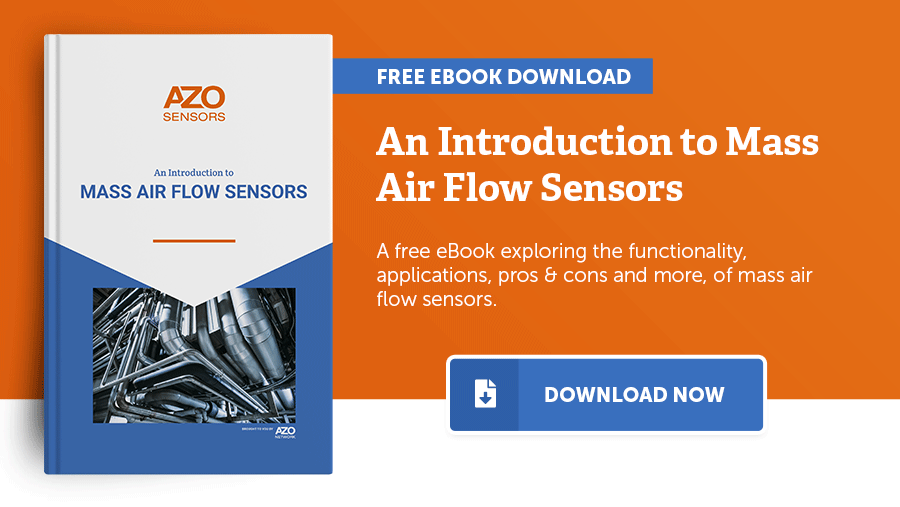Editorial Feature

# eBook: An Introduction to Mass Air Flow SensorsMass air flow sensors are a type of sensor used to measure mass air flow; working out the rate of gas passing through a given point at a given time.

There are a few subtle differences between mass air flow sensors and flow meters for measuring rates of gas flow. Mass air flow measurements specifically record the number of molecules in a flowing gas, as opposed to flow meters that measure the volume.

As gases are compressible, and their volumetric flow rates are sensitive to changes in pressure and temperature, mass air flow sensors are widely used for applications where it is necessary to know exactly how much gas there is, independent of environmental conditions.

Mass air flow sensors are commonly found in automotive, medical, and ventilation applications, and as a result, there are several commonly used designs of mass air flow sensors. Hot-wire or hot-film sensors, cold-wire sensors, and older designs such as Kármán vortex sensors, are all designs that have slightly different approaches to taking mass air flow measurements.

## Citations

• APA

AZoSensors. (2019, December 04). eBook: An Introduction to Mass Air Flow Sensors. AZoSensors. Retrieved on September 23, 2023 from https://www.azosensors.com/article.aspx?ArticleID=1745.

• MLA

AZoSensors. "eBook: An Introduction to Mass Air Flow Sensors". AZoSensors. 23 September 2023. <https://www.azosensors.com/article.aspx?ArticleID=1745>.

• Chicago

AZoSensors. "eBook: An Introduction to Mass Air Flow Sensors". AZoSensors. https://www.azosensors.com/article.aspx?ArticleID=1745. (accessed September 23, 2023).

• Harvard

AZoSensors. 2019. eBook: An Introduction to Mass Air Flow Sensors. AZoSensors, viewed 23 September 2023, https://www.azosensors.com/article.aspx?ArticleID=1745.

## Tell Us What You Think